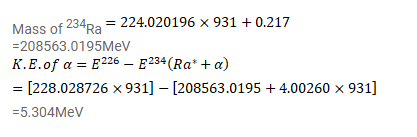# 228Th emits an alpha particle to reduce to 224Ra.

Question:

${ }^{228}$ Th emits an alpha particle to reduce to ${ }^{224} \mathrm{Ra}$. Calculate the kinetic energy of the alpha particle emitted in the following decay:

${ }^{228} \mathrm{Th} \rightarrow{ }^{224} \mathrm{Ra}{ }^{\star}+\alpha$

${ }^{224} \mathrm{Ra}^{*} \rightarrow{ }^{224} \mathrm{Ra}+\gamma(217 \mathrm{keV})$

Atomic mass of ${ }^{228} \mathrm{Th}$ is $228.028726 \mathrm{u}$, that of ${ }^{224} \mathrm{Ra}$ is $224.020196 \mathrm{u}$ and that of ${ }^{4}{ }_{2} \mathrm{He}$ is $4.00260 \mathrm{u}$.

Solution: